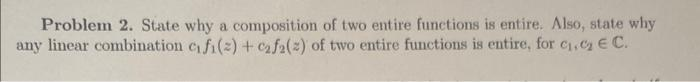# Question Solved1 AnswerAnswer Problem 2. Make sure its clear to read, Show all work. Problem 2. State why a composition of two entire functions is entire. Also, state why any linear combination $$c_{1} f_{1}(z)+c_{2} f_{2}(z)$$ of two entire functions is entire, for $$c_{1}, c_{2} \in \mathbb{C}$$.Answer Problem 2. Make sure its clear to read, Show all work.Transcribed Image Text: Problem 2. State why a composition of two entire functions is entire. Also, state why any linear combination $$c_{1} f_{1}(z)+c_{2} f_{2}(z)$$ of two entire functions is entire, for $$c_{1}, c_{2} \in \mathbb{C}$$.
More
Transcribed Image Text: Problem 2. State why a composition of two entire functions is entire. Also, state why any linear combination $$c_{1} f_{1}(z)+c_{2} f_{2}(z)$$ of two entire functions is entire, for $$c_{1}, c_{2} \in \mathbb{C}$$.